## Breeder

A pair of Positive Integers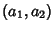such that the equationshave a Positive Integer solution, whereis the Divisor Function. Ifis Prime, then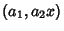is an Amicable Pair (te Riele 1986).is a special'' breeder if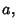whereandare Relatively Prime,. If regular amicable pairs of type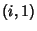withare of the form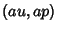with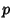Prime, thenare special breeders (te Riele 1986).

References

te Riele, H. J. J. Computation of All the Amicable Pairs Below.'' Math. Comput. 47, 361-368 and S9-S35, 1986.